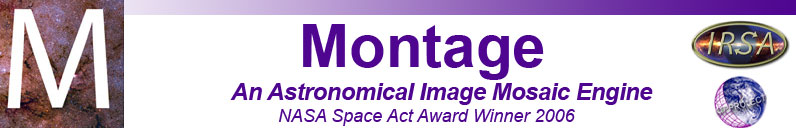# mBackground

Description:
Remove a background plane from a FITS image. The background correction applied to the image is specified as Ax+By+C, where (x,y) is the pixel coordinate using the image center as the origin, and (A,B,C) are the background plane parameters specified as linear coefficients.
Syntax:
mBackground [-d level] [-n(o-areas)] [-s statusfile] in.fits out.fits A B C
mBackground [-t] [-d level] [-n(o-areas)] [-s statusfile] in.fits out.fits images.tbl corrfile.tbl

Switches:

-d level
Turns on debugging to the specified level.
-n
Indicates that no area images are present (assumes equal weighting for each data pixel)
-s statusfile
mBackground output and errors will be written to statusfile instead of stdout.
-t
Run in table mode. Starts a group of mBackground runs in parallel, reading off the same pair of tables to determine which files and background corrections to work with. Recommended for running only on grids or parallel processors.

Arguments:

in.fits
Input FITS file
out.fits
Output FITS file
A B C
Corrections (as given by mFitplane or mFitExec)
images.tbl
When run as part of a GRID processing job, mBackground requires the image metadata table to retrieve the filenames of images.
corrections.tbl
When run as part of a GRID processing job, mBackground requires a table of corrections (from mFitplane and mFitExec) to apply to the corresponding image (from images.tbl).
Results:
Output FITS image with background subtraction applied.

Examples:

The following is sample output from mFitplane for a FITS image, in.fits:

[struct stat="OK", a=-0.000144415, b=-0.000133642, c=-31.8984, crpix1=758.5, crpix2=-1032, xmin=-758.5, xmax=-349.5, ymin=1032, ymax=1498, xcenter=-555.007, ycenter=1265.26, rms=0.576727]

We use the plane coefficients calculated by mFitplane to subtract the background from the image:

\$ mBackground projdir/in.fits bg_removed/in.fits -0.000144415 -0.000133642 -31.8984
[struct stat="OK"]

Return Codes:

• [struct stat = "OK"]
• [struct stat="ERROR", msg="Usage: mBackground [-d level] [-n(o-areas)] [-s statusfile] in.fits out.fits A B C | mBackground [-t] [-d level] [-n(o-areas)] [-s statusfile] in.fits out.fits images.tbl corrfile.tbl"]
• [struct stat="ERROR", msg="No status file name given"]
• [struct stat="ERROR", msg="Cannot open status file: statusfile"]
• [struct stat="ERROR", msg="No debug level given"]
• [struct stat="ERROR", msg="Debug level string is invalid: string"]
• [struct stat="ERROR", msg="Debug level value cannot be negative"]
• [struct stat="ERROR", msg="Invalid input file filename"]
• [struct stat="ERROR", msg="Invalid output file filename"]
• [struct stat="ERROR", msg="A coefficient string is not a number"]
• [struct stat="ERROR", msg="B coefficient string is not a number"]
• [struct stat="ERROR", msg="C coefficient string is not a number"]
• [struct stat="ERROR", msg="Invalid image metadata file: images.tbl"]
• [struct stat="ERROR", msg="Image table needs columns cntr and fname"]
• [struct stat="ERROR", msg="Hit end of image table without finding file name"]
• [struct stat="ERROR", msg="Need columns: id,a,b,c in corrections file"]
• [struct stat="ERROR", msg="Hit end of corrections table without finding data"]
• [struct stat="ERROR", status=error-code, msg="FITS library error message"]
• [struct stat="ERROR", msg="general error message"]